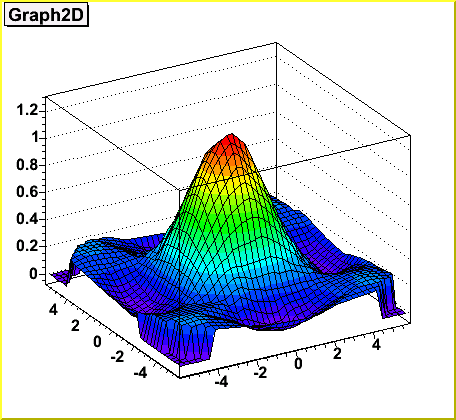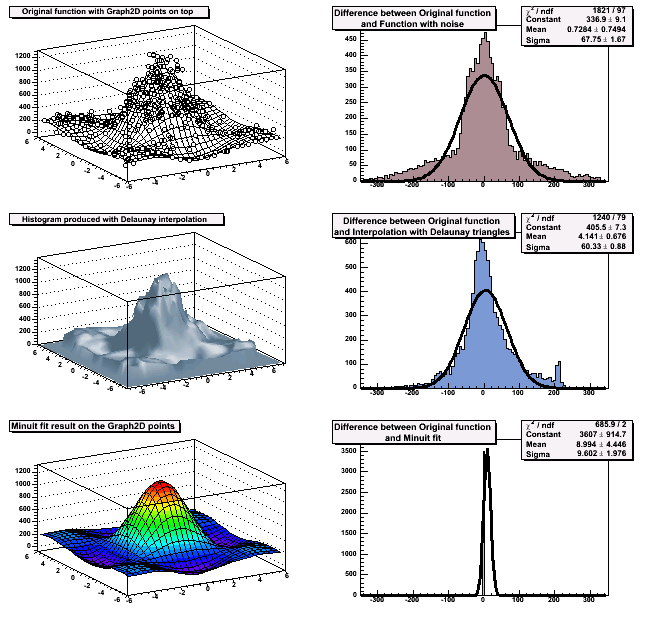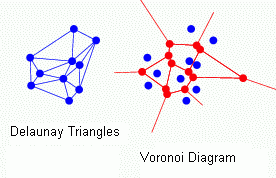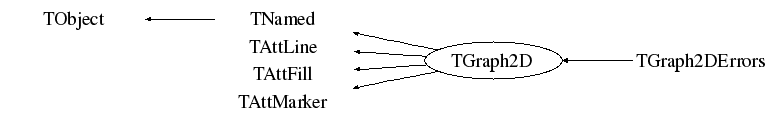# class TGraph2D: public TNamed, public TAttLine, public TAttFill, public TAttMarker

```
A Graph2D is a graphics object made of three arrays X, Y and Z with the same
number of points each.

This class has different constructors:

1) With an array's dimension and three arrays x, y, and z:

TGraph2D *g = new TGraph2D(n, x, y, z);

x, y, z arrays can be doubles, floats, or ints.

2) With an array's dimension only:

TGraph2D *g = new TGraph2D(n);

The internal arrays are then filled with SetPoint. The following line
fills the the internal arrays at the position "i" with the values x,y,z.

g->SetPoint(i, x, y, z);

3) Without parameters:

TGraph2D *g = new TGraph2D();

again SetPoint must be used to fill the internal arrays.

4) From a file:

TGraph2D *g = new TGraph2D("graph.dat");

Arrays are read from the ASCII file "graph.dat" according to a specifies
format. The format's default value is "%lg %lg %lg"

Note that in any of these three cases, SetPoint can be used to change a data
point or add a new one. If the data point index (i) is greater than the
current size of the internal arrays, they are automatically extended.

Specific drawing options can be used to paint a TGraph2D:
"TRI"  : The Delaunay triangles are drawn using filled area.
An hidden surface drawing technique is used. The surface is
painted with the current fill area color. The edges of each
triangles are painted with the current line color.
"TRIW" : The Delaunay triangles are drawn as wire frame
"TRI1" : The Delaunay triangles are painted with color levels. The edges
of each triangles are painted with the current line color.
"TRI2" : the Delaunay triangles are painted with color levels.
"P"    : Draw a marker at each vertex
"P0"   : Draw a circle at each vertex. Each circle background is white.

A TGraph2D can be also drawn with ANY options valid to draw a 2D histogram.

When a TGraph2D is drawn with one of the 2D histogram drawing option,
a intermediate 2D histogram is filled using the Delaunay triangles
technique to interpolate the data set.

TGraph2D linearly interpolate a Z value for any (X,Y) point given some
existing (X,Y,Z) points. The existing (X,Y,Z) points can be randomly
scattered. The algorithm works by joining the existing points to make
Delaunay triangles in (X,Y). These are then used to define flat planes
in (X,Y,Z) over which to interpolate. The interpolated surface thus takes
the form of tessellating triangles at various angles. Output can take the
form of a 2D histogram or a vector. The triangles found can be drawn in 3D.

This software cannot be guaranteed to work under all circumstances. They
were originally written to work with a few hundred points in an XY space
with similar X and Y ranges.

The picture below has been generated by the following macro:

{
TCanvas *c = new TCanvas("c","Graph2D example",0,0,700,600);
Double_t x, y, z, P = 6.;
Int_t np = 200;
TGraph2D *dt = new TGraph2D();
TRandom *r = new TRandom();
for (Int_t N=0; N<np; N++) {
x = 2*P*(r->Rndm(N))-P;
y = 2*P*(r->Rndm(N))-P;
z = (sin(x)/x)*(sin(y)/y)+0.2;
dt->SetPoint(N,x,y,z);
}
gStyle->SetPalette(1);
dt->Draw("surf1");
}

```
/**/
```
A more complete example can be find in \$ROOTSYS/tutorial/graph2dfit.C. It
produces the following output:

```
/**/
```
Definition of Delaunay triangulation (After B. Delaunay):
For a set S of points in the Euclidean plane, the unique triangulation DT(S)
of S such that no point in S is inside the circumcircle of any triangle in
DT(S). DT(S) is the dual of the Voronoi diagram of S.
If n is the number of points in S, the Voronoi diagram of S is the partitioning
of the plane containing S points into n convex polygons such that each polygon
contains exactly one point and every point in a given polygon is closer to its
central point than to any other. A Voronoi diagram is sometimes also known as
a Dirichlet tessellation.

```
/*This applet gives a nice practical view of Delaunay triangulation and Voronoi diagram. */

## Function Members (Methods)

public:
 TGraph2D() TGraph2D(Int_t n) TGraph2D(TH2* h2) TGraph2D(const TGraph2D&) TGraph2D(const char* filename, const char* format = "%lg %lg %lg", Option_t* option = "") TGraph2D(Int_t n, Int_t* x, Int_t* y, Int_t* z) TGraph2D(Int_t n, Float_t* x, Float_t* y, Float_t* z) TGraph2D(Int_t n, Double_t* x, Double_t* y, Double_t* z) TGraph2D(const char* name, const char* title, Int_t n, Double_t* x, Double_t* y, Double_t* z) virtual ~TGraph2D() void TObject::AbstractMethod(const char* method) const virtual void TObject::AppendPad(Option_t* option = "") virtual void TObject::Browse(TBrowser* b) static TClass* Class() virtual const char* TObject::ClassName() const virtual void TNamed::Clear(Option_t* option = "") virtual TObject* TNamed::Clone(const char* newname = "") const virtual Int_t TNamed::Compare(const TObject* obj) const virtual void TNamed::Copy(TObject& named) const virtual void TObject::Delete(Option_t* option = "") Int_t TAttLine::DistancetoLine(Int_t px, Int_t py, Double_t xp1, Double_t yp1, Double_t xp2, Double_t yp2) virtual Int_t DistancetoPrimitive(Int_t px, Int_t py) virtual void Draw(Option_t* option = "") virtual void TObject::DrawClass() const virtual TObject* TObject::DrawClone(Option_t* option = "") const virtual void TObject::Dump() const virtual void TObject::Error(const char* method, const char* msgfmt) const virtual void TObject::Execute(const char* method, const char* params, Int_t* error = 0) virtual void TObject::Execute(TMethod* method, TObjArray* params, Int_t* error = 0) virtual void ExecuteEvent(Int_t event, Int_t px, Int_t py) virtual void TObject::Fatal(const char* method, const char* msgfmt) const virtual void TNamed::FillBuffer(char*& buffer) virtual TObject* FindObject(const char* name) const virtual TObject* FindObject(const TObject* obj) const virtual Int_t Fit(const char* formula, Option_t* option = "", Option_t* goption = "") virtual Int_t Fit(TF2* f2, Option_t* option = "", Option_t* goption = "") TList* GetContourList(Double_t contour) TDirectory* GetDirectory() const virtual Option_t* TObject::GetDrawOption() const static Long_t TObject::GetDtorOnly() virtual Double_t GetErrorX(Int_t bin) const virtual Double_t GetErrorY(Int_t bin) const virtual Double_t GetErrorZ(Int_t bin) const virtual Double_t* GetEX() const virtual Double_t* GetEY() const virtual Double_t* GetEZ() const virtual Color_t TAttFill::GetFillColor() const virtual Style_t TAttFill::GetFillStyle() const TH2D* GetHistogram(Option_t* option = "") virtual const char* TObject::GetIconName() const virtual Color_t TAttLine::GetLineColor() const virtual Style_t TAttLine::GetLineStyle() const virtual Width_t TAttLine::GetLineWidth() const TList* GetListOfFunctions() const Double_t GetMargin() const virtual Color_t TAttMarker::GetMarkerColor() const virtual Size_t TAttMarker::GetMarkerSize() const virtual Style_t TAttMarker::GetMarkerStyle() const Int_t GetN() const virtual const char* TNamed::GetName() const Int_t GetNpx() const Int_t GetNpy() const virtual char* TObject::GetObjectInfo(Int_t px, Int_t py) const static Bool_t TObject::GetObjectStat() virtual Option_t* TObject::GetOption() const virtual const char* TNamed::GetTitle() const virtual UInt_t TObject::GetUniqueID() const Double_t* GetX() const TAxis* GetXaxis() const Double_t GetXmax() const Double_t GetXmin() const Double_t* GetY() const TAxis* GetYaxis() const Double_t GetYmax() const Double_t GetYmin() const Double_t* GetZ() const TAxis* GetZaxis() const Double_t GetZmax() const Double_t GetZmin() const virtual Bool_t TObject::HandleTimer(TTimer* timer) virtual ULong_t TNamed::Hash() const virtual void TObject::Info(const char* method, const char* msgfmt) const virtual Bool_t TObject::InheritsFrom(const char* classname) const virtual Bool_t TObject::InheritsFrom(const TClass* cl) const virtual void TObject::Inspect() const Double_t Interpolate(Double_t x, Double_t y) void TObject::InvertBit(UInt_t f) virtual TClass* IsA() const virtual Bool_t TObject::IsEqual(const TObject* obj) const virtual Bool_t TObject::IsFolder() const Bool_t TObject::IsOnHeap() const virtual Bool_t TNamed::IsSortable() const virtual Bool_t TAttFill::IsTransparent() const Bool_t TObject::IsZombie() const virtual void TNamed::ls(Option_t* option = "") const void TObject::MayNotUse(const char* method) const virtual void TAttLine::Modify() virtual Bool_t TObject::Notify() static void TObject::operator delete(void* ptr) static void TObject::operator delete(void* ptr, void* vp) static void TObject::operator delete[](void* ptr) static void TObject::operator delete[](void* ptr, void* vp) void* TObject::operator new(size_t sz) void* TObject::operator new(size_t sz, void* vp) void* TObject::operator new[](size_t sz) void* TObject::operator new[](size_t sz, void* vp) TGraph2D operator=(const TGraph2D&) virtual void Paint(Option_t* option = "") virtual void TObject::Pop() virtual void TNamed::Print(Option_t* option = "") const TH1* Project(Option_t* option = "x") const virtual Int_t TObject::Read(const char* name) virtual void TObject::RecursiveRemove(TObject* obj) Int_t RemovePoint(Int_t ipoint) virtual void TAttFill::ResetAttFill(Option_t* option = "") virtual void TAttLine::ResetAttLine(Option_t* option = "") virtual void TAttMarker::ResetAttMarker(Option_t* toption = "") void TObject::ResetBit(UInt_t f) virtual void TObject::SaveAs(const char* filename = "", Option_t* option = "") const virtual void TAttFill::SaveFillAttributes(ostream& out, const char* name, Int_t coldef = 1, Int_t stydef = 1001) virtual void TAttLine::SaveLineAttributes(ostream& out, const char* name, Int_t coldef = 1, Int_t stydef = 1, Int_t widdef = 1) virtual void TAttMarker::SaveMarkerAttributes(ostream& out, const char* name, Int_t coldef = 1, Int_t stydef = 1, Int_t sizdef = 1) virtual void SavePrimitive(ostream& out, Option_t* option = "") virtual void Set(Int_t n) void TObject::SetBit(UInt_t f) void TObject::SetBit(UInt_t f, Bool_t set) virtual void SetDirectory(TDirectory* dir) virtual void TObject::SetDrawOption(Option_t* option = "") static void TObject::SetDtorOnly(void* obj) virtual void TAttFill::SetFillAttributes() virtual void TAttFill::SetFillColor(Color_t fcolor) virtual void TAttFill::SetFillStyle(Style_t fstyle) virtual void SetHistogram(TH2* h) virtual void TAttLine::SetLineAttributes() virtual void TAttLine::SetLineColor(Color_t lcolor) virtual void TAttLine::SetLineStyle(Style_t lstyle) virtual void TAttLine::SetLineWidth(Width_t lwidth) void SetMargin(Double_t m = 0.1) void SetMarginBinsContent(Double_t z = 0.) virtual void TAttMarker::SetMarkerAttributes() virtual void TAttMarker::SetMarkerColor(Color_t tcolor = 1) virtual void TAttMarker::SetMarkerSize(Size_t msize = 1) virtual void TAttMarker::SetMarkerStyle(Style_t mstyle = 1) void SetMaximum(Double_t maximum = -1111) void SetMaxIter(Int_t n = 100000) void SetMinimum(Double_t minimum = -1111) virtual void SetName(const char* name) virtual void SetNameTitle(const char* name, const char* title) void SetNpx(Int_t npx = 40) void SetNpy(Int_t npx = 40) static void TObject::SetObjectStat(Bool_t stat) void SetPoint(Int_t point, Double_t x, Double_t y, Double_t z) virtual void SetTitle(const char* title = "") virtual void TObject::SetUniqueID(UInt_t uid) virtual void ShowMembers(TMemberInspector& insp, char* parent) virtual Int_t TNamed::Sizeof() const virtual void Streamer(TBuffer& b) void StreamerNVirtual(TBuffer& b) virtual void TObject::SysError(const char* method, const char* msgfmt) const Bool_t TObject::TestBit(UInt_t f) const Int_t TObject::TestBits(UInt_t f) const virtual void TObject::UseCurrentStyle() virtual void TObject::Warning(const char* method, const char* msgfmt) const virtual Int_t TObject::Write(const char* name = 0, Int_t option = 0, Int_t bufsize = 0) virtual Int_t TObject::Write(const char* name = 0, Int_t option = 0, Int_t bufsize = 0) const
protected:
 void Build(Int_t n) virtual void TObject::DoError(int level, const char* location, const char* fmt, va_list va) const void TObject::MakeZombie()

## Data Members

public:
 enum TObject::EStatusBits { kCanDelete kMustCleanup kObjInCanvas kIsReferenced kHasUUID kCannotPick kNoContextMenu kInvalidObject }; enum TObject::[unnamed] { kIsOnHeap kNotDeleted kZombie kBitMask kSingleKey kOverwrite kWriteDelete };
protected:
 TDirectory* fDirectory !Pointer to directory holding this 2D graph Color_t TAttFill::fFillColor fill area color Style_t TAttFill::fFillStyle fill area style TList* fFunctions Pointer to list of functions (fits and user) TH2D* fHistogram !2D histogram of z values linearly interpolated Color_t TAttLine::fLineColor line color Style_t TAttLine::fLineStyle line style Width_t TAttLine::fLineWidth line width Double_t fMargin Extra space (in %) around interpolated area for fHistogram Color_t TAttMarker::fMarkerColor Marker color index Size_t TAttMarker::fMarkerSize Marker size Style_t TAttMarker::fMarkerStyle Marker style Int_t fMaxIter Maximum number of iterations to find Delaunay t> Double_t fMaximum Maximum value for plotting along z Double_t fMinimum Minimum value for plotting along z TString TNamed::fName object identifier Int_t fNpoints Number of points in the data set Int_t fNpx Number of bins along X in fHistogram Int_t fNpy Number of bins along Y in fHistogram TVirtualHistPainter* fPainter !pointer to histogram painter Int_t fSize !Real size of fX, fY and fZ TString TNamed::fTitle object title Double_t* fX [fNpoints] Double_t* fY [fNpoints] Data set to be plotted Double_t* fZ [fNpoints] Double_t fZout fHistogram bin height for points lying outside the interpolated area
private:
 Bool_t fUserHisto True when SetHistogram has been called

## Class Charts## Function documentation

TGraph2D()
``` Graph2D default constructor
```
TGraph2D(Int_t n, Int_t *x, Int_t *y, Int_t *z)
``` Graph2D constructor with three vectors of ints as input.
```
TGraph2D(Int_t n, Float_t *x, Float_t *y, Float_t *z)
``` Graph2D constructor with three vectors of floats as input.
```
TGraph2D(Int_t n, Double_t *x, Double_t *y, Double_t *z)
``` Graph2D constructor with three vectors of doubles as input.
```
TGraph2D(TH2 *h2)
``` Graph2D constructor with a TH2 (h2) as input.
Only the h2's bins within the X and Y axis ranges are used.
```
TGraph2D(const char* name, const char* title, Int_t n, Double_t* x, Double_t* y, Double_t* z)
``` Graph2D constructor with name, title and three vectors of doubles as input.
name   : name of 2D graph (avoid blanks)
title  : 2D graph title
if title is of the form "stringt;stringx;stringy;stringz"
the 2D graph title is set to stringt, the x axis title to stringy,
the y axis title to stringy,etc
```

``` Graph2D constructor. The arrays fX, fY and fZ should be filled via
calls to SetPoint
```
TGraph2D(const char* filename, const char* format = "%lg %lg %lg", Option_t* option = "")
``` Graph2D constructor reading input from filename
filename is assumed to contain at least three columns of numbers
```
TGraph2D(const TGraph2D &g)
``` Graph2D copy constructor.
```

``` TGraph2D destructor.
```
TGraph2D operator=(const TGraph2D& )
``` Graph2D operator "="
```
void Build(Int_t n)
``` Creates the 2D graph basic data structure
```

``` Computes distance from point px,py to a graph
```
void Draw(Option_t* option = "")
``` Specific drawing options can be used to paint a TGraph2D:

"TRI"  : The Delaunay triangles are drawn using filled area.
An hidden surface drawing technique is used. The surface is
painted with the current fill area color. The edges of each
triangles are painted with the current line color.
"TRIW" : The Delaunay triangles are drawn as wire frame
"TRI1" : The Delaunay triangles are painted with color levels. The edges
of each triangles are painted with the current line color.
"TRI2" : the Delaunay triangles are painted with color levels.
"P"    : Draw a marker at each vertex
"P0"   : Draw a circle at each vertex. Each circle background is white.
"PCOL" : Draw a marker at each vertex. The color of each marker is
defined according to its Z position.
"CONT" : Draw contours
"LINE" : Draw a 3D polyline

A TGraph2D can be also drawn with ANY options valid to draw a 2D histogram.

When a TGraph2D is drawn with one of the 2D histogram drawing option,
a intermediate 2D histogram is filled using the Delaunay triangles
technique to interpolate the data set.
```
void ExecuteEvent(Int_t event, Int_t px, Int_t py)
``` Executes action corresponding to one event
```
TObject * FindObject(const char *name)
``` search object named name in the list of functions
```
TObject * FindObject(const TObject *obj)
``` search object obj in the list of functions
```
Int_t Fit(const char *fname, Option_t *option, Option_t *)
``` Fits this graph with function with name fname
Predefined functions such as gaus, expo and poln are automatically
created by ROOT.
fname can also be a formula, accepted by the linear fitter (linear parts divided
by "++" sign), for example "x++sin(y)" for fitting "*x+*sin(y)"
```
Int_t Fit(TF2 *f2, Option_t *option, Option_t *)
``` Fits this 2D graph with function f2

f2 is an already predefined function created by TF2.
Predefined functions such as gaus, expo and poln are automatically
created by ROOT.

The list of fit options is given in parameter option.
option = "W" Set all weights to 1; ignore error bars
= "U" Use a User specified fitting algorithm (via SetFCN)
= "Q" Quiet mode (minimum printing)
= "V" Verbose mode (default is between Q and V)
= "R" Use the Range specified in the function range
= "N" Do not store the graphics function, do not draw
= "0" Do not plot the result of the fit. By default the fitted function
is drawn unless the option"N" above is specified.
= "+" Add this new fitted function to the list of fitted functions
(by default, any previous function is deleted)
= "C" In case of linear fitting, not calculate the chisquare
(saves time)
= "ROB" In case of linear fitting, compute the LTS regression
coefficients (robust(resistant) regression), using
the default fraction of good points
"ROB=0.x" - compute the LTS regression coefficients, using
0.x as a fraction of good points

In order to use the Range option, one must first create a function
with the expression to be fitted. For example, if your graph2d
has a defined range between -4 and 4 and you want to fit a gaussian
only in the interval 1 to 3, you can do:
TF2 *f2 = new TF2("f2","gaus",1,3);
graph2d->Fit("f2","R");

Setting initial conditions

Parameters must be initialized before invoking the Fit function.
The setting of the parameter initial values is automatic for the
predefined functions : poln, expo, gaus. One can however disable
this automatic computation by specifying the option "B".
You can specify boundary limits for some or all parameters via
f2->SetParLimits(p_number, parmin, parmax);
if parmin>=parmax, the parameter is fixed
Note that you are not forced to fix the limits for all parameters.
For example, if you fit a function with 6 parameters, you can do:
func->SetParameters(0,3.1,1.e-6,0.1,-8,100);
func->SetParLimits(4,-10,-4);
func->SetParLimits(5, 1,1);
With this setup, parameters 0->3 can vary freely
Parameter 4 has boundaries [-10,-4] with initial value -8
Parameter 5 is fixed to 100.

Fit range

The fit range can be specified in two ways:
- specify rxmax > rxmin (default is rxmin=rxmax=0)
- specify the option "R". In this case, the function will be taken
instead of the full graph range.

Changing the fitting function

By default the fitting function Graph2DFitChisquare is used.
To specify a User defined fitting function, specify option "U" and
call the following functions:
TVirtualFitter::Fitter(mygraph)->SetFCN(MyFittingFunction)
where MyFittingFunction is of type:
extern void MyFittingFunction(Int_t &npar, Double_t *gin, Double_t &f, Double_t *u, Int_t flag);

Associated functions

One or more object (typically a TF2*) can be added to the list
of functions (fFunctions) associated to each graph.
When TGraph::Fit is invoked, the fitted function is added to this list.
Given a graph gr, one can retrieve an associated function
with:  TF2 *myfunc = gr->GetFunction("myfunc");

If the graph is made persistent, the list of
associated functions is also persistent. Given a pointer (see above)
to an associated function myfunc, one can retrieve the function/fit
parameters with calls such as:
Double_t chi2 = myfunc->GetChisquare();
Double_t par0 = myfunc->GetParameter(0); //value of 1st parameter
Double_t err0 = myfunc->GetParError(0);  //error on first parameter

Fit Statistics

You can change the statistics box to display the fit parameters with
the TStyle::SetOptFit(mode) method. This mode has four digits.
mode = pcev  (default = 0111)
v = 1;  print name/values of parameters
e = 1;  print errors (if e=1, v must be 1)
c = 1;  print Chisquare/Number of degress of freedom
p = 1;  print Probability

For example: gStyle->SetOptFit(1011);
prints the fit probability, parameter names/values, and errors.
You can change the position of the statistics box with these lines
(where g is a pointer to the TGraph):

Root > TPaveStats *st = (TPaveStats*)g->GetListOfFunctions()->FindObject("stats")
Root > st->SetX1NDC(newx1); //new x start position
Root > st->SetX2NDC(newx2); //new x end position
```

``` Get x axis of the graph.
```

``` Get y axis of the graph.
```

``` Get z axis of the graph.
```
TList * GetContourList(Double_t contour)
``` Returns the X and Y graphs building a contour. A contour level may
consist in several parts not connected to each other. This function
returns them in a graphs' list.
```
Double_t GetErrorX(Int_t bin) const
``` This function is called by Graph2DFitChisquare.
It always returns a negative value. Real implementation in TGraph2DErrors
```
Double_t GetErrorY(Int_t bin) const
``` This function is called by Graph2DFitChisquare.
It always returns a negative value. Real implementation in TGraph2DErrors
```
Double_t GetErrorZ(Int_t bin) const
``` This function is called by Graph2DFitChisquare.
It always returns a negative value. Real implementation in TGraph2DErrors
```
TH2D * GetHistogram(Option_t* option = "")
``` By default returns a pointer to the Delaunay histogram. If fHistogram
doesn't exist, books the 2D histogram fHistogram with a margin around
the hull. Calls TGraphDelaunay::Interpolate at each bin centre to build up
an interpolated 2D histogram.
If the "empty" option is selected, returns an empty histogram booked with
the limits of fX, fY and fZ. This option is used when the data set is
drawn with markers only. In that particular case there is no need to
find the Delaunay triangles.
```

``` Returns the X maximum
```

``` Returns the X minimum
```

``` Returns the Y maximum
```

``` Returns the Y minimum
```

``` Returns the Z maximum
```

``` Returns the Z minimum
```

``` Finds the z value at the position (x,y) thanks to
the Delaunay interpolation.
```
void Paint(Option_t* option = "")
``` Paints this 2D graph with its current attributes
```
TH1 * Project(Option_t* option = "x") const
``` Projects a 2-d graph into 1 or 2-d histograms depending on the
option parameter
option may contain a combination of the characters x,y,z
option = "x" return the x projection into a TH1D histogram
option = "y" return the y projection into a TH1D histogram
option = "xy" return the x versus y projection into a TH2D histogram
option = "yx" return the y versus x projection into a TH2D histogram
```
Int_t RemovePoint(Int_t ipoint)
``` Deletes point number ipoint
```
void SavePrimitive(ostream& out, Option_t* option = "")
``` Saves primitive as a C++ statement(s) on output stream out
```
void Set(Int_t n)
``` Set number of points in the 2D graph.
Existing coordinates are preserved.
New coordinates above fNpoints are preset to 0.
```
void SetDirectory(TDirectory* dir)
``` By default when an 2D graph is created, it is added to the list
of 2D graph objects in the current directory in memory.
Remove reference to this 2D graph from current directory and add
reference to new directory dir. dir can be 0 in which case the
2D graph does not belong to any directory.
```
void SetHistogram(TH2* h)
``` Sets the histogram to be filled
```
void SetMargin(Double_t m = 0.1)
``` Sets the extra space (in %) around interpolated area for the 2D histogram
```
void SetMarginBinsContent(Double_t z = 0.)
``` Sets the histogram bin height for points lying outside the TGraphDelaunay
convex hull ie: the bins in the margin.
```
void SetMaximum(Double_t maximum = -1111)
``` Set maximum.
```
void SetMinimum(Double_t minimum = -1111)
``` Set minimum.
```
void SetName(const char* name)
``` Changes the name of this 2D graph
```
void SetNameTitle(const char* name, const char* title)
``` Change the name and title of this 2D graph

```
void SetNpx(Int_t npx = 40)
``` Sets the number of bins along X used to draw the function
```
void SetNpy(Int_t npx = 40)
``` Sets the number of bins along Y used to draw the function
```
void SetPoint(Int_t point, Double_t x, Double_t y, Double_t z)
``` Sets point number n.
If n is greater than the current size, the arrays are automatically
extended.
```
void SetTitle(const char* title = "")
``` Sets graph title
```
void Streamer(TBuffer& b)
``` Stream a class object
```

`{return fDirectory;}`

`{return fNpx;}`

`{return fNpy;}`

`{ return fFunctions; }`

`{return fMargin;}`

`{return fNpoints;}`
Double_t * GetX()
`{return fX;}`
Double_t * GetY()
`{return fY;}`
Double_t * GetZ()
`{return fZ;}`

`{return 0;}`

`{return 0;}`

`{return 0;}`
void SetMaxIter(Int_t n = 100000)
`{fMaxIter = n;}`

Author: Olivier Couet
Last update: root/hist:\$Id: TGraph2D.h,v 1.00
Copyright (C) 1995-2000, Rene Brun and Fons Rademakers. *

This page has been automatically generated. If you have any comments or suggestions about the page layout send a mail to ROOT support, or contact the developers with any questions or problems regarding ROOT.